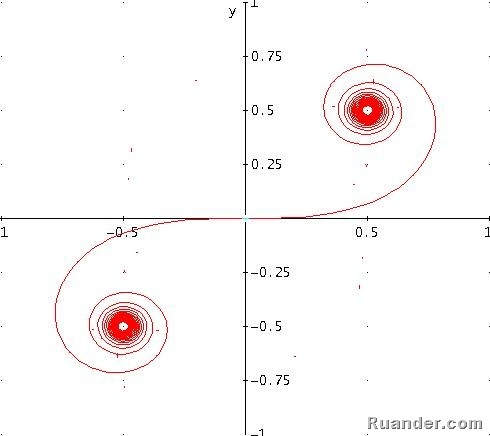## Pages

### Cornu's Spiral

It is very interesting to see the many different shapes that one can graph with a mathematical expression. Even more interesting is the idea that most of these mathematical expressions have been created for a reason. For example, let’s look at the following parametric equation know as the Cornu’s Spiral:If one were the graph the above parametric equation, the resultant figure would look as follow:Does anyone have an idea of what is the Cornu’s Spiral used for? Even if you don’t have any idea of what is it used for, it is very interesting to observe the type of figures you can obtain from an equation, isn’t?